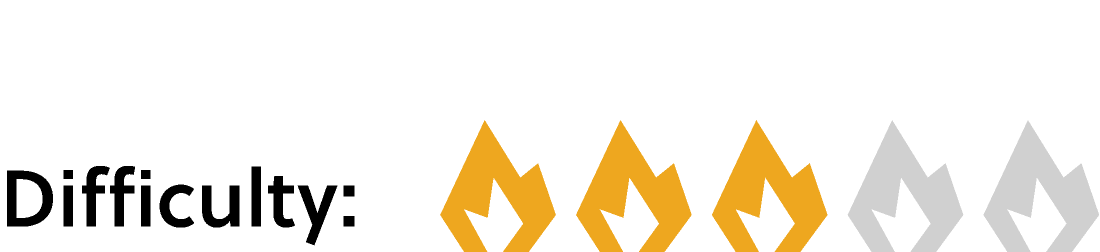## Cliquey Calcdoku

The goal of a $3\times 3$ Calcdoku puzzle is to fill the squares with a single digit each, with the following rules:

1. Each of the digits $1$ through $3$ is used exactly once in each row and column. (There is no region rule as in Sudoku.)

2. Any marked region indicates a number and an operation $(+,-,\times,$ or $\div).$ The operation applies to digits in the region and, when applied to the digits in some order, they result in the given number. In the example below, the region marked $“\, 7+"$ must be filled with three digits selected from $1$ through $3$ that have a sum of $7.$ Digits can be repeated in a marked region, but not in a row or column.

Keep reading to see two example puzzles, or jump straight to the daily challenge below.

To see solution steps for the $3\times 3$ puzzle below with underlying logic, use the right and left arrows below the puzzle:

The $4\times 4$ puzzle below is completed with the same rules, only now using digits $1$ through $4.$ Note how some regions are marked with operations other than addition, and also note that two squares are already filled with digits. Again, use the right and left arrows below the puzzle to see a solution method:

# Today's Challenge

When the puzzle is complete, what is the sum of the digits on the diagonal from the upper left to the lower right?